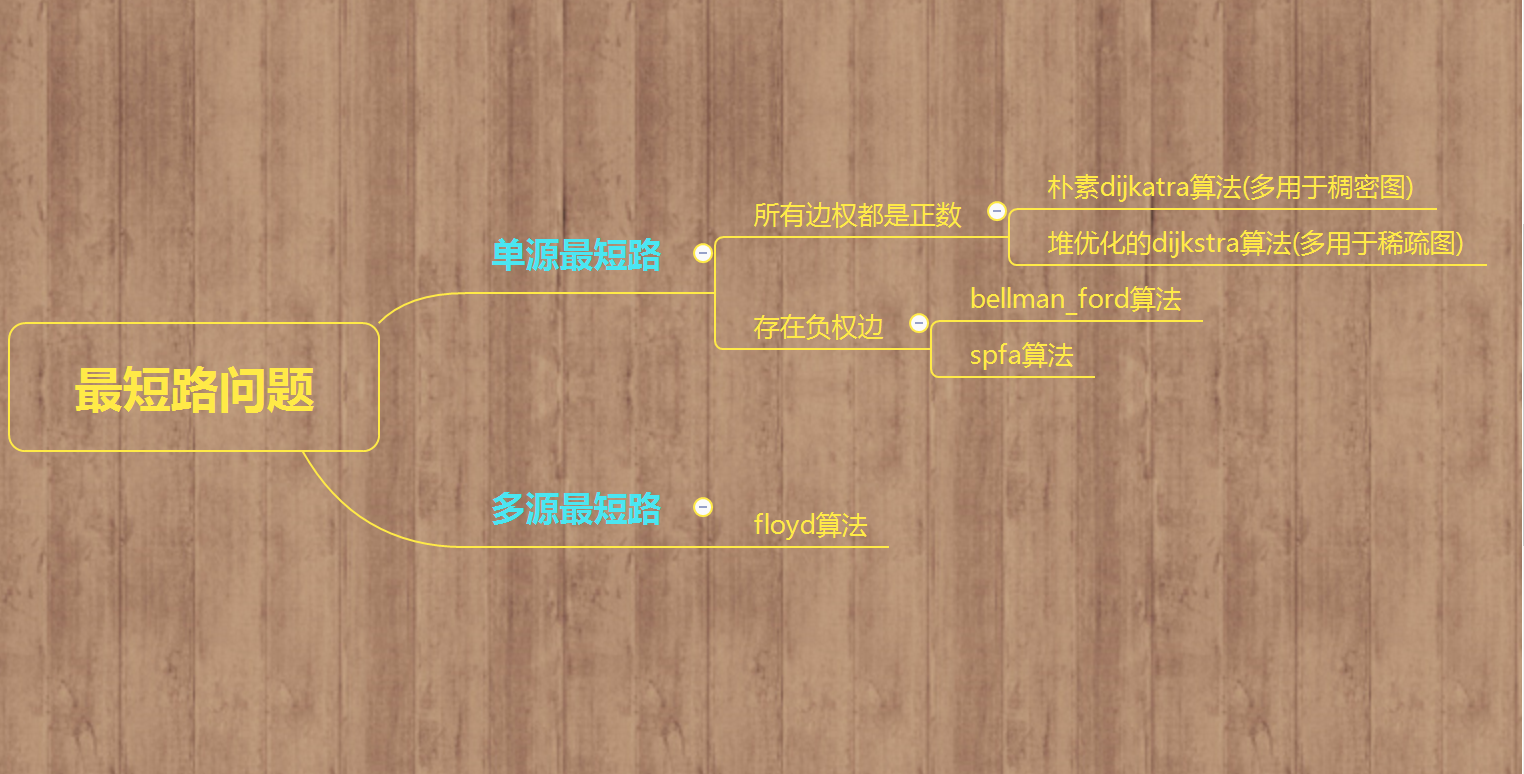• 请你计算从1号点到其他点的最短路。 输入描述: 第一行两个整数n, m。 接下来的m行，每行有三个整数u, v, l，表示u到v有一条长度为l的边。 输出描述: 共n-1行，第i行表示1号点到i+1号点的最短路。 输入 3 3 1 2...
链接：https://ac.nowcoder.com/acm/problem/14369
来源：牛客网
题目描述

简单暴力的题目要求：
给定一个有n个顶点（从1到n编号），m条边的有向图（其中某些边权可能为负，但保证没有负环）。请你计算从1号点到其他点的最短路。

输入描述:

第一行两个整数n, m。
接下来的m行，每行有三个整数u, v, l，表示u到v有一条长度为l的边。

输出描述:

共n-1行，第i行表示1号点到i+1号点的最短路。

输入

3 3
1 2 -1
2 3 -1
3 1 2

输出

-1
-2

解题思路
这道题用的方法是spfa+前向星图，存图然后遍历，再输出
解题代码
#include<bits/stdc++.h>
using namespace std;
int cnt,n,m;
int dist;
int flag;
queue<int> q;
struct Edge{
int v,next,w;
}edge;
cnt++;
edge[cnt].v = v;
edge[cnt].w = w;
}

void Spfa(int t)
{
memset(dist,127,sizeof(dist));
memset(flag,0,sizeof(flag));
dist[t]=0;
flag[t]=1;
q.push(t);
while(!q.empty()){
int v = q.front();
q.pop();
flag[v]=0;
if(dist[edge[i].v]>edge[i].w+dist[v]){
dist[edge[i].v]=edge[i].w+dist[v];
if(!flag[edge[i].v]){
q.push(edge[i].v);
flag[edge[i].v]=1;
}
}
}
}
for(int i=1;i<=n;i++){	//输出
if(i!=t){
cout<<dist[i]<<endl;
}
}
}

int main()
{
int u,v,w;
ios::sync_with_stdio(0);
cin.tie(0);cout.tie(0);
cin>>n>>m;
for(int i=1;i<=m;i++){
cin>>u>>v>>w;
}
Spfa(1);

}

说明

对于10%的数据，n = 2，m = 2。
对于30%的数据，n <= 5，m <= 10。
对于100%的数据，1 <= n <= 20000，1 <= m <= 200000，-10000 <= l <= 10000，保证从任意顶点都能到达其他所有顶点。


展开全文算法 数据结构
• 一提起最短路，各位oier会想到什么呢？ floyd，spfa，dij，或是bellman-ford？ 其实，只要学会一种算法，大部分最短路问题就能很快解决了。 他就是堆优化的dijkstra。 首先，先讲一下dij是怎么求最短路的。 ...
一提起最短路，各位oier会想到什么呢？
floyd，spfa，dij，或是bellman-ford？
其实，只要学会一种算法，大部分最短路问题就能很快解决了。
他就是堆优化的dijkstra。
首先，先讲一下dij是怎么求最短路的。
Dijkstra是基于一种贪心的策略，首先用数组dis记录起点到每个结点的最短路径，再用一个数组保存已经找到最短路径的点
然后，从dis数组选择最小值，则该值就是源点s到该值对应的顶点的最短路径，并且把该点记为已经找到最短路
此时完成一个顶点，再看这个点能否到达其它点（记为v），将dis[v]的值进行更新
不断重复上述动作，将所有的点都更新到最短路径
这种算法实际上是O(n^2)的时间复杂度，但我们发现在dis数组中选择最小值时，我们可以用一些数据结构来进行优化。
其实我们可以用STL里的堆来进行优化，堆相对于线段树以及平衡树有着常数小，码量小等优点，并且堆的一个妙妙的性质就是可以在nlogn的时限内满足堆顶是堆内元素的最大（小）值，之不正是我们要的嘛？
但是呢，dij处理不了负边，所以当题目出现负边时，dij就不能用了。
但反过来说，只要题目没负边，SPFA是一定会被卡的！
下面上代码：

#include<bits/stdc++.h>
using namespace std;
#define maxn 10005
#define maxm 500005
#define INF  1234567890
{
int x=0,k=1; char c=getchar();
while(c<'0'||c>'9'){if(c=='-')k=-1;c=getchar();}
while(c>='0'&&c<='9')x=(x<<3)+(x<<1)+(c^48),c=getchar();
return x*k;
}
struct Edge
{
int u,v,w,next;
}e[maxm];
struct node
{
int w,now;
inline bool operator <(const node &x)const
//重载运算符把最小的元素放在堆顶（大根堆）
{
return w>x.w;//这里注意符号要为'>'
}
};
priority_queue<node>q;
//优先队列，其实这里一般使用一个pair，但为了方便理解所以用的结构体
inline void add(int u,int v,int w)
{
e[++cnt].u=u;
//这句话对于此题不需要，但在缩点之类的问题还是有用的
e[cnt].v=v;
e[cnt].w=w;
//存储该点的下一条边
//更新目前该点的最后一条边（就是这一条边）
}
//链式前向星加边
void dijkstra()
{
for(int i=1;i<=n;i++)
{
dis[i]=INF;
}
dis[s]=0;
//赋初值
q.push((node){0,s});
while(!q.empty())
//堆为空即为所有点都更新
{
node x=q.top();
q.pop();
int u=x.now;
//记录堆顶（堆内最小的边）并将其弹出
if(vis[u]) continue;
//没有遍历过才需要遍历
vis[u]=1;
//搜索堆顶所有连边
{
int v=e[i].v;
if(dis[v]>dis[u]+e[i].w)
{
dis[v]=dis[u]+e[i].w;
//松弛操作
q.push((node){dis[v],v});
//把新遍历到的点加入堆中
}
}
}
}
int main()
{
for(int i=1,x,y,z;i<=m;i++)
{
}
dijkstra();
for(int i=1;i<=n;i++)
{
printf("%d ",dis[i]);
}
return 0;
}

谢谢大家！
转载于:https://www.cnblogs.com/mxrmxr/p/9851795.html
展开全文• ## C++最短路算法

千次阅读 2018-06-01 20:56:51
最短路第一步肯定是在输入的时候建边 然后搜索一次，最后输出最优解 一般用数组存，和dp很类似 言归正传，这一道题有2种方法 1：SPFA（宽搜） a[x][y]记录点x到y的路径（直接，联系，一开始初始化为无限大） d[x]...
题目描述【题意】给出一个图，起始点是1，结束点是N，边是双向的。求点1到点N的最短距离。哈哈，这就是标准的最短路径问题。 【输入格式】第一行为两个整数N（1≤N≤10000）和M（0≤M≤200000）。N表示图中点的数目，M表示图中边的数目。下来M行，每行三个整数x，y，c表示点x到点y之间存在一条边长度为c。（x≠y,1≤c≤10000）【输出格式】输出一行，一个整数，即为点1到点N的最短距离。如果点1和点N不联通则输出-1。【样例1输入】2 11 2 3【样例1输出】3【样例2输入】3 31 2 52 3 53 1 2【样例2输出】2【样例3输入】6 91 2 71 3 91 5 142 3 102 4 153 4 113 5 24 6 65 6 9【样例3输出】20这就是最基本的最短路问题（caioj.cn1088）最短路第一步肯定是在输入的时候建边然后搜索一次，最后输出最优解一般用数组存，和dp很类似言归正传，这一道题有2种方法1：SPFA（宽搜）a[x][y]记录点x到y的路径（直接，联系，一开始初始化为无限大）d[x]记录从1到点x的最小距离v[i]记录点i是否在宽搜队列里面输入：scanf("%d%d%d",&x,&y,&c);
if(c<a[x][y]) a[x][y]=c,a[y][x]=c;初始化：memset(d,127,sizeof(d));d=0;//先变得无限大，方便以后，点1自己到自己的距离为0
memset(v,false,sizeof(v));v=true;//一开始只有点1在队列里面 主要程序：memset(d,127,sizeof(d));d=0;//先变得无限大，方便以后，点1自己到自己的距离为0
memset(v,false,sizeof(v));v=true;//一开始只有点1在队列里面
{
for(y=1;y<=n;y++)
{
if(a[x][y]<=10000)//题目说<=10000，表明这是一条边
{
if(d[y]>d[x]+a[x][y])//如果以前到达这一个点的最小值比这一种方案要大
{
d[y]=d[x]+a[x][y];//更新
if(v[y]==false)//如果不在队列里面（剪枝），在对列里面的直接更新就可以了
{
v[y]=true;//把它标记为“在”
list[tail]=y;//放进队列里面
tail++;if(tail==n+1) tail=1;//队列尾+1，最后一句是为了循环利用空间，节省内存
}
}
}
}
v[y]=false;//出队列
}如果觉得这样比较慢，我们可以加一个目录，虽然麻烦一点点，可是快了很多先定义一个结构体struct node
{
int x,y,d,next;//x和y表示两个点，d表示费用，next表示以x开头的下一条边的编号（最后结束就是next=0，表示下一条没有边）
}a;int len,last;
//len表示边的数量
//last表示以x开头最后一条边的编号建边函数inline void ins(int x,int y,int d)
{
len++;
a[len].x=x;a[len].y=y;a[len].d=d;
a[len].next=last[x];last[x]=len;
}记得建双向边(ins(x,y);ins(y,x))找边就是:for(int k=last[x];k>0;k=a[k].next)2、Floyd（3重循环，时间o(n^3））先定义一个inf（无穷大），把每一条边设置为inf，输入完以后标准的五行代码：for(int i=1;i<=n;i++)
for(int j=1;j<=n;j++)
for(int k=1;k<=n;k++)
if(d[i][j]>d[i][k]+d[k][j])
d[i][j]=d[i][k]+d[k][j];注这个代码时间很长，边多的时候不要用
展开全文• 先上本篇博客的主要内容(图片有一处打错了应该是dijkstra) 朴素dijkstra算法 时间复杂度为O(n2)，多用于不存在负权边的稠密图(指边数远大于节点数)中 int g[N][N], dis[N], n...//储存每个点的最短路是否确定 int ...
先上本篇博客的主要内容(图片有一处打错了应该是dijkstra)朴素dijkstra算法
时间复杂度为O(n2)，多用于不存在负权边的稠密图(指边数远大于节点数)中
int g[N][N], dis[N], n, m;//g[N][N]为邻接矩阵用于存储每条边，dis[N]存储某个点到1号点的最小距离， n为点数，m为边数
bool visit[N];//储存每个点的最短路是否确定

int dijkstra()
{
memset(dis, 0x3f, sizeof(dis));
dis = 0;

for(int i = 0; i < n - 1; i++)
{
int t = -1;//在未确定最短路的点中寻找距离一号店点最近的点
for(int j = 1; j <= n; j ++)
{
if(!visit[j] && (t == -1 || dis[t] > dis[j]))
t = j;
}

visit[t] = true;
//用t更新其他点的距离
for(int j = 1; j <= n; j++)
{
dis[j] = min(dis[j], dis[t] + g[t][j]);
}
}
return dis[n];
}

堆优化版dijkstra算法
时间复杂度为(mlogn)，多用于不存在负权边且为稀疏图(边数约等于节点数)中
typedef pair<int, int> P;
int h[N], ne[N], e[N], w[N], idx;//用邻接表来储存边
int n, m, dis[N];//储存所有点到一号点的距离
bool visit[N];//判断每个点的最短路是否确定

void add(int a, int b, int c)//将边添加到邻接表中
{
e[idx] = b, w[idx] = c, ne[idx] = h[a], h[a] = idx++;
}

int dijkstra(void)
{
memset(dis, 0x3f, sizeof(dis));
dis = 0;

priority_queue<P, vector<P>, greater<P> > heap;
heap.push({0, 1});//first储存距离， second储存编号

while(!heap.empty())
{
P t = heap.top();
heap.pop();

int ver = t.second, distance = t.first;
if(visit[ver]) continue;
visit[ver] = true;

for(int i = h[ver]; i != -1; i = ne[i])
{
int j = e[i];
if(dis[j] > distance + w[i])
{
dis[j] = distance + w[i];
heap.push({dis[j], j});
}
}
}
return dis[n];
}

bellman_ford算法
时间复杂度为O(nm)，用于存在负权边的最短路径，也可存在负权回路
int n, m;       // n表示点数，m表示边数
int dist[N];        // dist[x]存储1到x的最短路距离

struct Edge     // 边，a表示出点，b表示入点，w表示边的权重
{
int a, b, w;
}edges[M];

// 求1到n的最短路距离，如果无法从1走到n，则返回-1。
int bellman_ford()
{
memset(dist, 0x3f, sizeof dist);
dist = 0;

// 如果第n次迭代仍然会松弛三角不等式，就说明存在一条长度是n+1的最短路径，由抽屉原理，路径中至少存在两个相同的点，说明图中存在负权回路。
for (int i = 0; i < n; i ++ )
{
for (int j = 0; j < m; j ++ )
{
int a = edges[j].a, b = edges[j].b, w = edges[j].w;
if (dist[b] > dist[a] + w)
dist[b] = dist[a] + w;
}
}

if (dist[n] > 0x3f3f3f3f / 2) return -1;
return dist[n];
}

spfa算法
最常用的最短路算法之一，时间复杂度为O(m)，最坏情况下为O(nm)
int h[N], w[N], ne[N], e[N], idx;//用邻接表储存边
int n, m, dis[N];//储存每个点到1号点距离
bool visit[N];//判断每个点是否在队列中

void add(int a, int b, int c)
{
e[idx] = b, w[idx] = c, ne[idx] = h[a], h[a] = idx++;
}

int spfa(void)
{
memset(dis, 0x3f, sizeof(dis));
dis = 0;

queue<int> heap;
heap.push(1);
while(!heap.empty())
{
int t = heap.front();
heap.pop();

visit[t] = false;
for(int i = h[t]; i != -1; i = ne[i])
{
int j = e[i];
if(dis[j] > dis[t] + w[i])
{
dis[j] = dis[t] + w[i];
if(!visit[j])
{
heap.push(j);
visit[j] = true;
}
}
}
}
return dis[n];
}

flody算法
时间复杂度为O(n3)，用于求多源最短路问题
int dis[N][N], n, m, k;

int floyd()
{
for(int k = 1; k <= n; k++)
{
for(int i = 1; i <= n; i++)
{
for(int j = 1; j <= n; j++)
{
dis[i][j] = min(dis[i][j], dis[i][k] + dis[k][j]);
}
}
}
}

本文章素材全部来自于AcWing的yxc大佬，AcWing网站
个人学习使用


展开全文• 最短路是个老问题了，大神们留下很多文档但是很多都是针对算法使用一些固定大小的数组进行数据存储在实际应用中受到限制，这里自己练习一下，主要用了一些c++的stl，减少了固定长度数组的依赖，换一种写法试图提高...
• 全源最短路问题。 即 对给定的图(V,E)，求任意两点u,v之间的最短路径长度（时间复杂度为O（n3）,所以顶点数n小于200） 实现方法 邻接矩阵 基本思想 若存在 dis[i][k] + dis[k][j]<dis[i][j] 则令 dis[i][j]=dis[i...算法
• C++6.0编的Dijkstra求最短路，解压就可以用。初始数据用矩阵表示已经在程序里了，改改就能用
• ## 最短路计数- C++

千次阅读 2020-02-02 21:58:51
最短路计数 题目描述 给出一个 N 个顶点 M 条边的无向无权图，顶点编号为1−N。问从顶点1开始，到其他每个点的最短路有几条。 输入格式 第一行包含2个正整数N,M，为图的顶点数与边数。 接下来M行，每行2个正整数x...dfs
• 我们在生活中常常遇到最短路问题，这些问题都可以抽象成图论中的最短路问题。Dijkstra算法可以用于解决这一类最短路问题，它可以实现计算权值为正的有向图中一个点到所有点的最小路径。dijkstra 算法
• #include #include using namespace std; const int &INF=100000000; void floyd(vector<vector<int> > &distmap,//可被更新的邻接矩阵，... cout短距离为"[beg][end]，打印路径："; print(beg,end,path); }
• 题目：P1144 最短路计数 他们居然说是水题，看来我还是太low了。 用spfa求最短路，然后记录有多少个相同的到该点的最短路。 你忽略了重边了吗？ # include # include # include using namespace std; const ...算法
• 1、单源最短路算法  n个处理器，第一个处理器要广播消息到其他所有的处理器，求需要时间最短是多少（从第一个点出发，求到其他点最短路的最大值） 2、思路  这个基本上没啥可说。看代码理解就是。 3、代码实现...
• Bellman_ford算法 #include<iostream> using namespace std; int d; int graph={{0,2,5,0,0,0,0},{2,0,4,6,10,0,0},{5,4,0,2,0,0,0},{0,6,2,0,0,1,0},{0,10,0,0,0,3,5},{0,0,0,1,3,0,9},{0,0,0,0,5,...算法
• 摘要:VC/C++源码,算法相关,队列优化,最短路径算法　C++队列优化的Bellmanford最短路算法（SPFA），使用C++实现的Queue improved Bellman-Ford单源最短路算法，在国内还被叫做SPFA。这个程序输入一个图，找到图中的一...
• 最短路是图论最重要的算法之一，也是算法难点。经过这篇的学习，你会发现你离成功就差一个最短路的距离。=.= 最短路是指，某个点到某个点之间的距离最短。 算法1：Dijkstra算法 迪杰斯特拉算法是很典型的单源最短路...算法
• (C++) BFS求解最短路长度及最短路路径 一、问题描述：（迷宫问题） 在一张由0， 1构成的图中，1表示障碍，0表示通路 给定起点S和终点T 求从S到T的最短路长度并输出路径 二、求解思路：（BFS） 首先BFS是由队列实现...BFS
• PAT(Advanced)甲级1030 Travel Plan Dijkstra最短路 C++实现 相关内容 PAT(Advanced)1003 Emergency (Dijkstra最短路) （邻接矩阵）C++实现 DijkstraSSSP（单源最短路径求解）（迪克斯特拉算法堆优化）（Java实现）...算法 dijkstra 贪心算法 数据结构
• 本程序是使用c++编写的最短路算法，需要输入的是一个graph的阶数以及赋权矩阵
• #include //输入一个矩阵数组，求出从arr到arr[row - 1][col - 1]的最短路径 int minPathSum(int (*arr), const int &row, const int &col); //数组并不完全是指针，传参数需要注意 ...动态规划 dp 矩阵...

# c++最短路c++ 订阅